### A Tutorial on Introductory Modern Physics: Fission, Fusion and Radioactivity

Target Audience: High School Students, College Freshmen and Sophomores, students preparing for the International Baccalaureate (IB), AP Physics B, AP Physics C, A Level, Singapore/GCE A-Level;

### This might also be helpful in studying topics required by Common Core Physics.

This compilation of notes has been prepared by Anirud Thyagarajan of IIT Kharagpur.

### NUCLEAR FISSION

The breaking of a heavy nucleus into two or more fragments of comparable masses, with the release of tremendous energy is called as nuclear fission. The most typical fission reaction occurs when slow moving neutrons strike 92U235. The following nuclear reaction takes place.

If more than one of the neutrons produced in the above fission reaction are capable of inducing a fission reaction (provided U235 is available), then the number of fissions taking place at successive stages goes increasing at a very brisk rate and this generates a series of fissions. This is known as chain reaction. The chain reaction takes place only if the size of the fissionable material (U235) is greater than a certain size called the critical size.

92U235 + 0n1 ——> 56Ba141 + 36Kr92 + 3 0n1 + 200 MeV

If the number of fission in a given interval of time goes on increasing continuously, then a condition of explosion is created. In such cases, the chain reaction is known as uncontrolled chain reaction. This forms the basis of atomic bomb.

In a chain reaction, the fast moving neutrons are absorbed by certain substances known as moderators (like heavy water), then the number of fissions can be controlled and the chain reaction is such cases is known as controlled chain reaction. This forms the basis of a nuclear reactor.

### NUCLEAR FUSION

The process in which two or more light nuclei are combined into a single nucleus with the release of tremendous amount of energy is called as nuclear fusion. Like a fission reaction, the sum of masses before the fusion (i.e. of light nuclei) is more than the sum of masses after the fusion (i.e. of bigger nucleus) and this difference appears as the fusion energy. The most typical fusion reaction is the fusion of two deuterium nuclei into helium.

1H1 + 1H2 —> 2He4 + 21.6 MeV

For the fusion reaction to occur, the light nuclei are brought closer to each other (with a distance of 10–14 m). This is possible only at very high temperature to counter the repulsive force between nuclei. Due to this reason, the fusion reaction is very difficult to perform. The inner core of sun is at very high temperature, and is suitable for fusion, in fact the source of sun's and other star's energy is the nuclear fusion reaction.

The dependence of radioactivity on other factors is also considered imperative.

It was observed by Henri Becquerel in 1896 that some minerals like pitchblende emit radiation spontaneously, and this radiation can blacken photographic plates if photographic plate is wrapped in light proof paper. He called this phenomenon radioactivity. It was observed that radioactivity was not affected by temperature, pressure or chemical combination, it could therefore be a property that was intrinsic to the atom-more specifically to its nucleus. Detailed studies of radioactivity resulted in the discovery that radiation emerging from radioactive substances were of three types:α-rays,  β-rays and γ-rays.

α-rays were found to be positively charged, β-rays (mostly) negatively charged, and γ-rays uncharged. Some of the properties of α, β and γ–rays, are explain below:

#### (iii) Gamma Rays:   γ-rays are electromagnetic waves of wavelength of the order of ~ 10–12 m. These have the maximum penetrating power (even more than X–ray) and the least ionizing power. These are emitted due to transition of excited nuclei from higher energy state to lower energy state.

Soddy, Fajan and Rutherford formulated rules of alpha and beta decay of atoms. When a nuclide emits an alpha particle, the mass number decreases by 4, and the atomic no. of the daughter nuclide decreases by 2. When a nuclide emits a beta particle, the mass number remains the same, with the atomic no. of the daughter nuclide increasing by one. Problems related to these concepts should be practiced well.

The number of atoms disintegrating per second γ is very small in the SI system it take a large number N(~ Avogadro number, 1023) to get any significant activity.

dN/dt = – γN               ….(i)

Where  γ is the radioactivity decay constant.

If No is the number of radioactive atoms present at a time t = 0, and N is the number at the end of time t, then

N = N0e– γt

The term (–dN/dt) is called the activity of a radioactive substance and is denoted by 'A'.

Units:

1 Becquerel (Bq) = 1 disintegration per second (dps)

1 curie (Ci)         = 3.7 x 1010 dps

1 Rutherford       =106 dps

The changes in N, due to radioactivity, are very small in 1 s compared with the value of N it’s self.

Therefore, N may be approximated by means of a continuous function N(t).

For the case considered in equation (i), we can write,

A = rate of decrease of N = λN

or  –dN/dt = λN                                       ..... (ii)

Note the equation (ii) is valid only for large value of N, typically –1018 or so.

If the number of atoms at time t = 0 (initial) is N = N0, then one can solve equation (ii):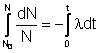or     In N/N0 = –λt

or     N(t) = N0 e–λt                                        ......(iii)

The activity A (t) = λN(t) and is given by

A (t) =λN0

= A0 λN0)           .... (iv)

MODERN PHYSICS: A Mix of Simple and Reasonably Challenging Problems

Simple Questions

1. A bulb emits light of mean wavelength 4500 A. The lamp is rated as 150 W an 8% of the energy appears as emitted light. How many photons are emitted by the lamp per second.

Solution: 8% of 150 W = P = 12 W

# of photons per sec =  P*/ h* c = 2.715 * 10^19. .. (Ans.)

2. If the intensity of a light source is increased, it necessarily follows that

a) there is an increase in the kinetic energies of the emitted photons

b) the wavelength has decreased

c) the linear momentum carried by the photons has increased

d) the photon emission rate has increased.

Solution: With increase in the intensity of the incident light, there occurs an increase in the no. of incident photons, thereby increasing the photon emission rate. Hence, the option is (d).

3. An electron in the ground state of hydrogen has an angular momentum L1, and an electron in the first excited state of lithium has an angular momentum L2. then

a) L1 = L2

b) L1 = 4L2

c) L2 = 2L1

d) L1 = 2L2

Solution: Using Bohr’s Quantisation Rule,

L1 = 1(h)/2

L2 = 2(h)/2

Therefore, L2 = 2L1, option C is the ans.

4. 1 gm of a radioactive material having half life period of 2 years is kept in store for a duration of 4 years. Calculate how much of the material remains unchanged ?

Solution : We know N = N0/2^n

where N0 ---- initial concentration

n ------ no. of half lives

n = 4/2 = 2 half lives

Thus, N = N0/2^2 = N0/4 = 0.25 gms.

5. A 280 days old radioactive substance shows an activity of 6000 dps. 140 days later its activity becomes 3000 dps. What was its initial activity?

Solution:   A1 = A0 * e^(-t)

A2 = A0 * e^(-(t+t1))

Using A1/A2 = e^(t1)

Using A1 = 6000  A2 = 3000 and t1 = 140, t = 280, we get

=>  = ln 2 / 140.

Thus, A0 = 24000.

6. Photoelectric effect is observed in a photosensisitive substance irradiated by a point source emitting uv light. As the photo-sensitive substance is moved away from the source,

a) the stopping potential decreases

b) the stopping potential increases

c) the photocurrent increases

d) the photocurrent decreases

Solution: Increasing the distance of the photosensitive metal from the source, decreases the intensity, which affects only the number of photoelectrons ejected per second, which constiutes the photocurrent. (d)

Moderately Difficult Questions

1. Light of wavelength 1800 A ejects photoelectrons from a plate of a metal whose work function is 2 eV. If a uniform magnetic field of 5 * 10^ -5 T is applied parallel to the plate, what would be the radius of the path followed by electrons ejected normally from the plate with maximum energy?

Solution: As calculated ,

E = 12431/1800 = 6.9 eV.

KE(max) = 6.9 - 2 = 4.9 eV.

and,

0.5 m*v^2 = 4.9 eV  => v = 1.31 * 10^6 m/s.

As we know when a charged particle enters a magnetic field at right angles, it moves in a circular path radius of which is given by

R = mv/qB = 0.149 m.

2. A laser beam  = 633 nm has a power of 3mW. Find the pressure exerted on a surface by this beam if the cross sectional area is 3mm^2. Assume perfect reflection at normal incidence.

Solution:

Q3. An ion with mass no. 56 contains 30 mts +ve charge & 30.4% more neutrons than electrons. What is symbol of ion?

Ans: Suppose no. of electrons in ion M3+ = x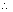No. of neutrons = x +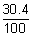x = 1.304 x

No. of electrons in neutral atom = x + 3No. of protons = x + 3

Mass no. = No. of photons + No. of neutrons

56 = x + 3 + 1.304 x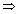x = 23

No. of protons = 23 + 3 = 26

Symbol of will be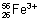Q4. Find velocity (m/s) of electron in Ist Bohr orbit of radius a0. Find be Broglie wavelength (in m). Find angular momentum of 2p orbital of H-atom.

Ans: For H & H-like atoms,

vn = 2.188 x 106 x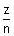m/s

For H-atom, z =1   & for Ist orbit   n = 1v = 2.188 x 106

Debroglie wavelength,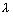=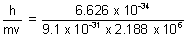= 3.33 x 10-10 m

Orbital angular momentum =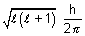For   2p,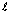= 1,

Orbital angular momentum =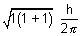=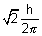Q5. A dye absorbs light of= 4530 A0 & then fluorescence light of 50870 A0. 47% of absorbed energy is reemitted out as flurescence. Calculate ratio of quanta emitted out to no. of quanta absorbed.

Let n1 photons areabsorbed.absorbed

Total energy absorbed =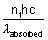E of light re-emitted out in one photon =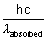Let n2 photons are reemitted then,

Total energy reemitted out =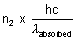As given

Eabsorbed x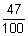= Ereemitted outx= n2 x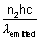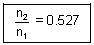Q6. What H-like ion has wavelength difference b/w Ist lines of Balmer & Lyman series equal to 59.3 nm   RH = 109678 cm-1

Ans: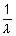= RHz2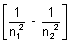For I line of Balmer series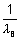= RHz2x RH x z2B =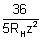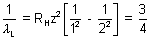RHzL =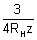B -L = 59.3 x 10-7 cm.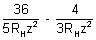= 59.3 x 10-7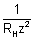[7.2 - 1.333] = 59.3 x 10-7

z2 =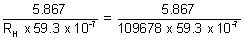z = 3

H-like atom is Li2+.

Q6. 1.8g H - atoms are excited to radiation. Spectra shows that 27% of atoms are in IIIrdenergy level & 1.5% of atoms in IInd energy level & rest in ground state. Ionization potential of H is 13.6 ev. Calculate

(i) No. of atoms present in III, II energy level.

Ans: 1g H contains = No atoms

1.8g H contains = 6.023 x 1023 x 1.8

= 10.84 x 1023 atoms

(a)No. of atoms in III shell =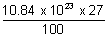= 292.68 x 1021 atoms.

=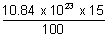= 162.6 x 1021 atoms.

(b) When all atoms return ti I shell, then

E' = (E3 - E1) x 292.68 x 1021

En = -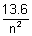ev

E' = (-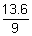+ 13.6) x 292.68 x 1021 x 1.6 x 10-19     [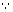1 ev = 1.6 x 10-19 J]

= 5.668 x 105 J

E'' = (E2 - E1) x 162.6 x 1021

= (-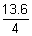+ 13.6) x 1.6 x 10-19 x 162.6 x 1021

= 2.657 x 105 J

E = E' + E'' = (5.668 + 2.657) x 105 J

Total energy evolved = 832.5 KJ

Q7. Find quantum no. 'n' corresponding to excited state of He+ ion if on transitiontoground state that ion emits two photons in succession with wavelengths 18.5 & 30.4 nm respectively.

Ans: Suppose electron in excited state is present in n2 shell. First it falls from n2 to n1 & then from n1 to ground state (n = 1).

(i) n2n11 = 108.5 nm

(ii) n1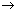G.State,2 = 30.4 nm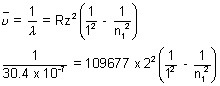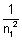= 1 - 0.75n12 =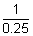n1 = 2

Again,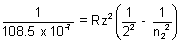= 109677 x 22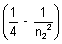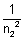= 0.25 - 0.21 = 0.04

n22 =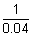= 25n2 = 5

Q8. The wave function of orbital is given by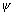(r) =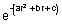. Find thevalue of r for which probability of finding electron is max.

Ans: Probability of finding electron2(r) =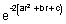For max. probability finding electron&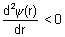..................= e-c[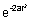(-4ar)e-2br +e-2br(-2b)]

= e-ce-2br[- 4ar - 2b][- 4ar - 2b] = 0

4ar = - 2b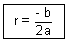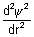= (- a)+ (- 4ar - 2b)2=[- 4a + (4ar + 2b)2]

=[- 4a + 16a2r2 + 4b2 + 16abr]

Putting   r = -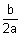=[- 4a + 16a2 x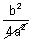+ 4b2 + 16ab x -]

=[- 4a +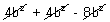]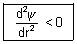Max. prob. of finding electron

when   r = -